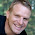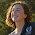# Basics of Electrical Engineering

Learn the basics of Electrical Engineering.

Engineering involves a lot of Mathematics, in fact, it is based on the mathematics. Along with many other rules and principles, the trigonometric identities are basic and fundamental equations every engineer should remember.

Today Basics of Electrical Engineering shares basic trigonometric identities. Let's revise the basic concepts below:

1.2.